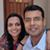D

#### Problem on calculating probability

31 viewed last edited 1 year agoAnonymous
0

I know that there are 24 ways of distributing 4 cards across 4 boxes. Don't know how to do the rest.

Suppose that 4 cards labeled 1 to 4 are placed randomly into 4 boxes also labeled 1 to 4, one card per box. What is the probability that no card gets placed into a box having the same label as the card?Vivekanand Vellanki
3

Let P be the probability that no card gets placed into a box with the same label.

P=P_0=1-\left(P_4+P_3+P_2+P_1\right) where P_nrepresents the probability that exactly n cards are placed in a box with the same label.

Finding P_4, etc is easier than finding P_0.

Finding P_4: All 4 cards are placed in a box with the same label. This can happen exactly once. So, P_4=\frac{1}{24}.

Finding P_3: Exactly 3 cards are placed in a box with the same label. This implies that the 4th card should also be placed in a box with the same label. Hence, it is not possible for exactly 3 cards to be placed in a box with the same label. So, P_3=\frac{0}{24}

Finding P_2: Exactly 2 cards are placed in a box with the same label. The remaining 2 cards should not be placed in a box with the same label.

There are 6 ways of picking the 2 numbers to place in a box with the same label. For each of the 6 ways, there is exactly one way in which the remaining 2 cards are placed into a box of different label. So, P_2=\frac{6}{24}.

Finding P_1: Exactly 1 card must be placed in a box with the same label. There are 4 ways of picking this 1 card.

For each such combination, each of the remaining 3 cards have to be placed in a box of different card. Let the 3 cards be named A, B and C. Pick the card A and place it in the box named B. Now, pick the card that does not correspond to the box in which A is placed - in this case C. This can be placed only in box A; and the last card B can be placed in box C. One combination is (A, B); (C, A) and (B, C). Similarly, if A is placed in box C, the combination is (A, C); (B, A) and (C, B)

For each combination, there are 2 ways in which all remaining cards are placed in boxes with a different label.

So, P_1=\frac{\left(4\cdot2\right)}{24}

Hence, P_0=1-\left(\frac{1}{24}+\frac{0}{24}+\frac{6}{24}+\frac{8}{24}\right)=1-\frac{15}{24}=\frac{9}{24}1

Here's an interesting meta-tip for problems like this - it's often easier to use negative, rather than positive counting. You'll see that the specific and general answers above use negative counting (count items that *do not* match the specification, then subtract from the total).

Why do this? Because with such problems, it's hard to make sure you don't double count. Try a positive count of the number of ways to distribute cards that match the problem spec, and you'll see how much harder it is.Mahesh Godavarti
0

Let's do it with recursion.

Let N_n = the number of ways n cards can be placed in such a way so that no card gets placed into a box having the same label as the card.

First, we can easily see that N_1 = 0 and N_2 = 1 .

Now, we will show that N_n = n! - \sum_{k=1}^{n-1} C(n, k) N_{n - k} - C(n, n) .

1. The first term in the expression n! is the total number of ways n labels can be arranged in n boxes.
2. Each term in the summation C(n, k) N_{n-k} denotes the total number of ways we can have only k of the cards in a matching box while the rest are not. We can obviously do it by first selecting k cards that need to be placed in a matching box and then arranging the remaining n - k cards in a mismatched box in all possible ways. For each selection of k cards, there are N_{n-k} ways of arranging the rest in mismatched boxes (this follows directly from the definition of N_n ). Since there are C(n, k) distinct ways of selecting k cards out of n , the total number of ways is C(n, k) \cdot N_{n-k} .
3. The last term denotes the total number of ways all n cards can be placed in their matching box which is obviously just 1 or C(n, n) .

Note that if we define N_0 = 1 then we can rewrite the expression in a much more compact form as follows:

N_n=n!-\sum_{k=1}^nC(n,k)N_{n-k}.

Therefore, we have N_3=3!-C(3,1)N_2-C(3,2)N_1-C(3,3)=6-3-0-1=2.

We have N_4=4!-C(4,1)N_3-C(4,2)N_2-C(4,3)N_1-C(4,4)=24-4\cdot2-6\cdot1-4\cdot0\ -1=24-8-6-0-1=9.

Therefore, the probability is \frac{N_4}{4!} = \frac{9}{24} .